Turnerj I wear glasses so I can C#.

# Levenshtein Distance (Part 2: Gotta Go Fast)

Feb 13, 2020

In Part 1 I explained what the Levenshtein Distance is and that it is both computationally and memory inefficient in a simple form. In Part 2 (this post), I'll cover ways to decrease the memory overhead and increase the performance.

## Example Levenshtein Implementation

Before we get started, I'll walk through a real implementation of Levenshtein Distance with no optimizations - this is what we will be improving from.

Note: While the examples in this post are in C#, there is very little that couldn't be copied over to any other programming language with only minor edits.

``````public int CalculateDistance(string source, string target)
{
var costMatrix = Enumerable
.Range(0, source.Length + 1)
.Select(line => new int[target.Length + 1])
.ToArray();

for (var i = 1; i <= source.Length; ++i)
{
costMatrix[i] = i;
}

for (var i = 1; i <= target.Length; ++i)
{
costMatrix[i] = i;
}

for (var i = 1; i <= source.Length; ++i)
{
for (var j = 1; j <= target.Length; ++j)
{
var insert = costMatrix[i][j - 1] + 1;
var delete = costMatrix[i - 1][j] + 1;
var edit = costMatrix[i - 1][j - 1] + (source[i - 1] == target[j - 1] ? 0 : 1);

costMatrix[i][j] = Math.Min(Math.Min(insert, delete), edit);
}
}

return costMatrix[source.Length][target.Length];
}
``````

Breaking this down:

``````var costMatrix = Enumerable
.Range(0, source.Length + 1)
.Select(line => new int[target.Length + 1])
.ToArray();
``````

This builds our matrix - an array of arrays - to be one longer than the source string (eg. "Sunday") in rows and one longer than the target string (eg. "Saturday") in columns.

``````for (var i = 1; i <= source.Length; ++i)
{
costMatrix[i] = i;
}

for (var i = 1; i <= target.Length; ++i)
{
costMatrix[i] = i;
}
``````

These two for-loops build the top row and left column of our matrix. With the example "Saturday" and "Sunday", it would look like this: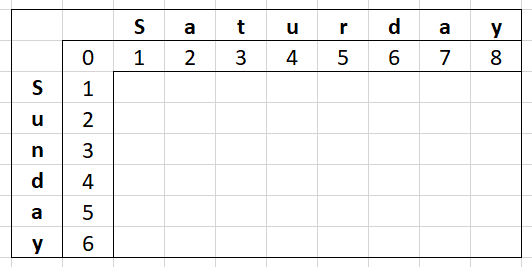We have our main code:

``````for (var i = 1; i <= source.Length; ++i)
{
for (var j = 1; j <= target.Length; ++j)
{
var insert = costMatrix[i][j - 1] + 1;
var delete = costMatrix[i - 1][j] + 1;
var edit = costMatrix[i - 1][j - 1] + (source[i - 1] == target[j - 1] ? 0 : 1);

costMatrix[i][j] = Math.Min(Math.Min(insert, delete), edit);
}
}
``````

Simply put - for every column in every row, check the cell to my left (insert), the cell above (delete) and the cell diagonally to the left (edit). We do our Levenshtein Distance math (explained in Part 1) and we store that in the current cell.

And finally, our humble return of the last cell in the matrix - our Levenshtein Distance.

``````return costMatrix[source.Length][target.Length];
``````

Now let's start making this faster and more memory efficient!

## Memory Optimizations

### Turning `(n+1)*(m+1)` into `(n+1)*2`

One of the biggest problems is the sheer amount of memory a full matrix of two long strings would require BUT it isn't necessary and here is why: We don't actually need all the data all the time.

Using same example we had in Part 1 with "Saturday" and "Sunday", yellow is the values we depend on and green is the values we calculate AND depend on.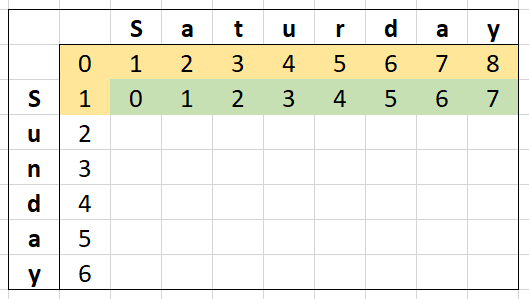If we continue this for the next row, it looks like this: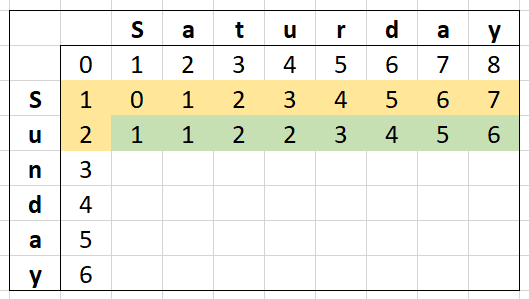We can see that we no longer depend on the very first row of the matrix so in reality, we only need two rows of memory.

Below is an example of how that might look in code:

``````public int CalculateDistance(string source, string target)
{
var costMatrix = Enumerable
.Range(0, 2)
.Select(line => new int[target.Length + 1])
.ToArray();

for (var i = 1; i <= target.Length; ++i)
{
costMatrix[i] = i;
}

for (var i = 1; i <= source.Length; ++i)
{
costMatrix[i % 2] = i;

for (var j = 1; j <= target.Length; ++j)
{
var insert = costMatrix[i % 2][j - 1] + 1;
var delete = costMatrix[(i - 1) % 2][j] + 1;
var edit = costMatrix[(i - 1) % 2][j - 1] + (source[i - 1] == target[j - 1] ? 0 : 1);

costMatrix[i % 2][j] = Math.Min(Math.Min(insert, delete), edit);
}
}

return costMatrix[source.Length % 2][target.Length];
}
``````

So what is going on here?

• Our `costMatrix` variable is nearly instantiated the same however we only are creating two rows.
• We dropped one of our for-loops, the one that builds the left column values. Instead, we now have `costMatrix[i % 2] = i;` in our main loop that effectively mimics this behaviour.
• We are using `i % 2` in a lot of places, allowing us to switch which row we are on.

That last point about `% 2` switching what row we are on might sound odd so let me explain. The `%` operator is called the "Modulo" operator - it gets the remainder after a division.

• If `i = 0`, the remainder of dividing by 2 is `0`
• If `i = 1`, the remainder of dividing by 2 is `1`
• If `i = 2`, the remainder of dividing by 2 is `0`
• and so on...

It is a nice little shortcut for us to switch between the two rows of data as if we had a full matrix.

The `source.Length % 2` on the return will always get us the "last" row.

So at this point, we've dramatically decreased the memory usage but know we can do better - what if we only needed one row...

### Turning `(n+1)*2` into `n+1`

I said earlier that we don't need all the data all the time and that is still the case with the two-row example above - we don't need all the data. In this case, we don't need all the data in the same row.

How does that work? Let's look at the matrix again...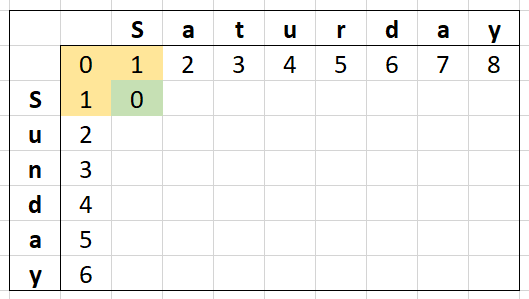With the yellow cells being what we depend on and green being what we calculated, we are only depending on 3 values. The next cell will also depend on only 3 values...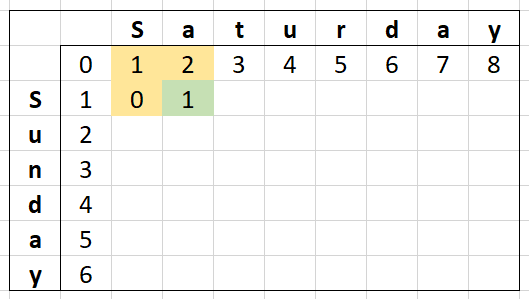Now repeating this for one more cell, we can see more clearly the values we don't care about any more for this row.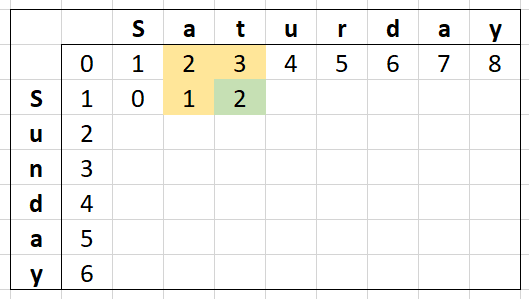When calculating the third cell, we don't actually care about the first cell we calculated. Taking this in mind, an implementation might look like this:

``````public int CalculateDistance(string source, string target)
{
var previousRow = new int[target.Length + 1];

for (var i = 1; i <= target.Length; ++i)
{
previousRow[i] = i;
}

for (var i = 1; i <= source.Length; ++i)
{
var previousDiagonal = previousRow;
var previousColumn = previousRow++;

for (var j = 1; j <= target.Length; ++j)
{
var insertOrDelete = Math.Min(previousColumn, previousRow[j]) + 1;
var edit = previousDiagonal + (source[i - 1] == target[j - 1] ? 0 : 1);

previousColumn = Math.Min(insertOrDelete, edit);
previousDiagonal = previousRow[j];
previousRow[j] = previousColumn;
}
}

return previousRow[target.Length];
}
``````

There are a lot more changes here and now with different variable names so let's break this down:

``````var previousRow = new int[target.Length + 1];
``````

Our `costMatrix` is out for a single array called `previousRow` - this will still hold our costs but from the point of view of our calculation, they will always be the "previous" set of data.

We still have our for-loop setting the first row like normal.

Our main loops are the other big differences:

``````for (var i = 1; i <= source.Length; ++i)
{
var previousDiagonal = previousRow;
var previousColumn = previousRow++;

for (var j = 1; j <= target.Length; ++j)
{
var insertOrDelete = Math.Min(previousColumn, previousRow[j]) + 1;
var edit = previousDiagonal + (source[i - 1] == target[j - 1] ? 0 : 1);

previousColumn = Math.Min(insertOrDelete, edit);
previousDiagonal = previousRow[j];
previousRow[j] = previousColumn;
}
}
``````

Starting with `previousDiagonal`, this represents our last substitution/edit cost. At the start of each logical row, the cost here is always one less than the insert cost (our `previousColumn`).

Speaking of `previousColumn`, with it being our last insert cost and knowing that our insert costs start one higher than our substitution/edit cost, we add one to the value of the `previousDiagonal`.

Our delete costs for our current column are found in `previousRow[j]` which also coincides with the value we are about to set.

In the meat of the for-loops, we can see I'm playing around with the variables a bit setting the `previousColumn` and `previousDiagonal` before finally setting `previousRow[j]` - this is all to make sure we have the right values in the right spots.

• We set `previousColumn` to be our result because our result will be the "previous" result for the next column over.
• We set `previousDiagonal` to be our our delete cost (`previousRow[j]`) as for the next column, our delete cost is their substitution/edit cost.

Finally after all the processing is done - we return the last value of our `previousRow`.

Now `n+1` is great but what if we wanted `n`-sized array instead? I can say it is totally possible with a bit more footwork with what variables you set where but I'll leave that up to the reader to work out. (Or stay tuned for Part 3 where we push things to 11)

## Performance/Time Optimizations

Now while the memory optimizations are great and will increase performance through less memory lookups or even from the removal of one of the for-loops, there are still big performance-specific optimizations on the table.

There are some basic shortcuts to the Levenshtein Distance, these include:

• If `StringA` is empty, the Levenshtein Distance is `StringB`'s length.
• If `StringB` is empty, the Levenshtein Distance is `StringA`'s length.
• If `StringA` is equal to `StringB`, the Levenshtein Distance is `0`.

Those are good but we can do better - What if we didn't even need build a matrix of all the letters from both words? What if we could trim the words that share a prefix or suffix? Now we're onto something...

With our example strings `Saturday` and `Sunday`, they share a common prefix and suffix so makes a great example for this. We only need to start where the strings are different and end on the last difference.

This makes "Saturday"-"Sunday" become "atur"-"un", a much smaller amount to process and as a bonus, a much smaller amount of memory needed!

Just to be sure it works, let's look at a matrix of this: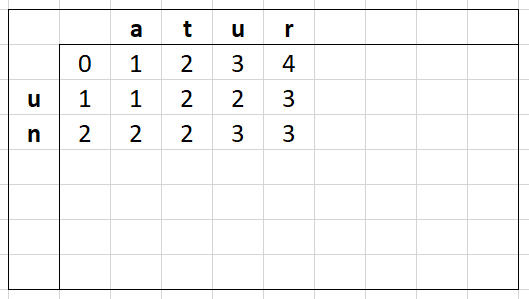We can actually find this segment in the full matrix of "Saturday" and "Sunday":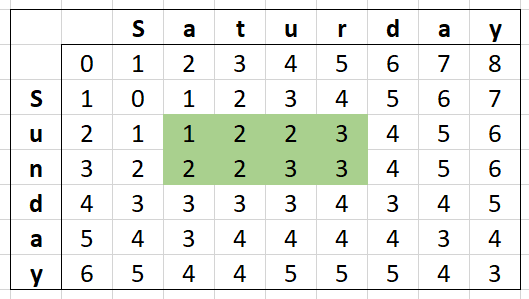This optimization can be extremely useful on large strings, bringing them down to a far more manageable size.

A partial implementation of this might look like:

``````var startIndex = 0;
var sourceEnd = source.Length;
var targetEnd = target.Length;

while (startIndex < sourceEnd && startIndex < targetEnd && source[startIndex] == target[startIndex])
{
startIndex++;
}
while (startIndex < sourceEnd && startIndex < targetEnd && source[sourceEnd - 1] == target[targetEnd - 1])
{
sourceEnd--;
targetEnd--;
}

var sourceLength = sourceEnd - startIndex;
var targetLength = targetEnd - startIndex;

source = source.Substring(startIndex, sourceLength);
target = target.Substring(startIndex, targetLength);
``````

## Gotta Go Faster - I want more optimizations!

So do I! In Part 3, we will take everything we've done to 11! We will remove any memory allocations we have, decrease the number of string lookups, use hardware intrinsics to improve performance, learn the secrets of loop unrolling and even dabble in parallel processing! 😈

It's gonna be great!